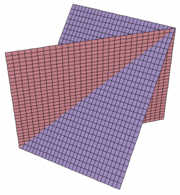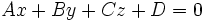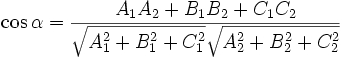# Dihedral Angle CalculatorA dihedral angle is the angle between two planes. To calculate this angle, you can follow these steps: 1. Calculate the equation for each plane. It will be in the form:2. Then, knowing the equation of the two planes, you can calculate the dihedral angle:I made this calculator to calculate the equation of each planes from three points, and then the angle between them: